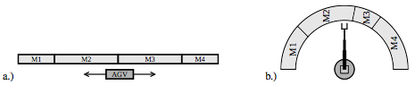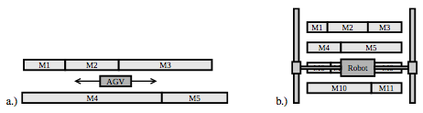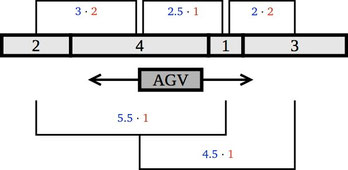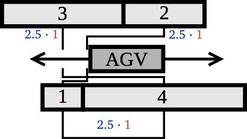# Who can quiet the animal shelter?

## Single- and Multi-Row Facility Layout Problem

The general facility layout design problem is concerned with placing departments of given areas within a given facility. To each possible placement is assigned a cost based on the interactions between each pair of departments. These costs reflect an appropriate measure of adjacency preferences between departments. In some versions of the problem, the dimensions of the departments are also given. When this is not the case, finding their optimal shape is also a part of the problem.

Versions of the facility layout problem occur in many practical contexts, not only in the planning of production and logistics but also in applications such as VLSI chip design.

In our interactive presentations we aim to explain a special version of the general facility layout problem, namely the Single- and Multi-Row Facility Layout Problem. It consists of a set of rectangular facilities, a given number of rows, and weights for each pair of departments. Now the aim is to find an assignment of departments to rows and the positions of the departments in each row so that the total weighted sum of the center-to-center distances between all pairs of departments is minimized.In a.) an AGV transports parts between the machines moving in both directions along a straight line. In b.) a material-handling industrial robot carries parts between the machines.In a.) an AGV transports parts between the machines that are located on both sides of a linear path of travel. In b.) a gantry robot is used when the space is limited.

Let us further clarify the workings the Single- and Multi-Row Facility Layout Problem with the help of a toy example: We consider 4 departments with lengths 1, 2, 3 and 4 respectively . Additionally we are given the pairwise connectivities c(1,2) = c(1,4) = c(3,4) = 1, c(1,3) = c(2,4) = 2. The figures below illustrate the optimal layouts and the associated costs for our problem with 1 and 2 rows respectively:Here we display the optimal layout for the Single-Row Facility Layout Problem with corresponding total cost of 22.5.Here we depict the optimal layout for the Multi-Row Facility Layout Problem with 2 rows with associated cost of 7.5.

We created a little game about the Single-Row Facility Layout Problem: